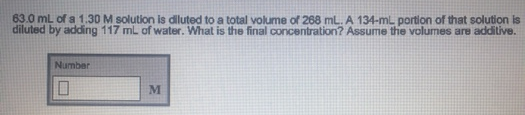# Problem: 63.0 mL of a 1.30 M solution is diluted to a total volume of 268 mL. A 134 mL portion of that solution is diluted by adding 117 mL of water. What is the final concentration? Assume the volumes are additive.

###### FREE Expert Solution
95% (208 ratings)###### Problem Details

63.0 mL of a 1.30 M solution is diluted to a total volume of 268 mL. A 134 mL portion of that solution is diluted by adding 117 mL of water. What is the final concentration? Assume the volumes are additive.